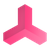0
Declined

# Force Torque Sensor

VIZtech4 8 months ago in General Questions updated 7 months ago

Hello,

Have you any plans to produce a Force Torque Sensor ?

Ideally it would be as simple as adding it as a component to say the mounting flange of a 6 axis robot to give information about the load.

I looked at the Force Drive but can't get sensible results from it.

Regards

DougHi, for our use cases we don't have the need so far for doing this calculation. I don't think that we are going to implement this in the near future. You could implement what you described, our source code is open for our customers.Under review

Hi, as far as we know measuring forces is with Unity PhysX engine not possible. You might need to use extended Physics Engines like Algoryx AGX but in this cases the drives also need to be force controlled, which is mostly not the case if you would like to position a drive exactly in simulation based on a calculated or transfered value from an industrial controller.Declined+1

Relative to a 6DOF robot arm, we know this information;

1. The velocity, axis, direction and angle of the Drive at each joint

2. The kinematic parameters describing the dimensions of the robot

It should therefore be possible to calculate the velocity of the TCP in each world axis on a frame by frame basis.

Then, using the Unity FixedUpdate() method it would be possible to calculate acceleration over each fixed time frame.

If the robot then picked up a MU where it's mass is known, using,  force = mass * acceleration, the forces in XYZ could be calculated each frame.

If you could do the maths for that it would be excellent.

I realise this measures only forces due to load but in a simulation this would most likely be adequate, it certainly would for our application.Hi, for our use cases we don't have the need so far for doing this calculation. I don't think that we are going to implement this in the near future. You could implement what you described, our source code is open for our customers.If you have joint angles, it's well doable:

1. Create DH matrixes and calculate Forward Kinematics

2. Calculate the TCP speed and the time span between two frames. Can you do something similar to this post from the ROS forum+1